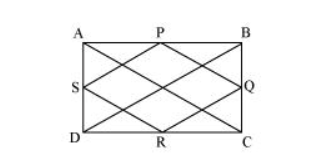# ABCD is a rectangle and P, Q, R and S are mid-points of the sides AB, BC, CD and DA respectively.Question. ABCD is a rectangle and P, Q, R and S are mid-points of the sides AB, BC, CD and DA respectively. Show that the quadrilateral PQRS is a rhombus.

Solution:Let us join $\mathrm{AC}$ and $\mathrm{BD}$.

In $\triangle \mathrm{ABC}$,

$P$ and $Q$ are the mid-points of $A B$ and $B C$ respectively.

$\therefore P Q \| A C$ and $P Q=\frac{1}{2} A C$ (Mid-point theorem) $\ldots$(1)

Similarly in $\triangle \mathrm{ADC}$,

$S R \| A C$ and $S R=\frac{1}{2} A C$ (Mid-point theorem) $\ldots$(2)

Clearly, $P Q \| S R$ and $P Q=S R$

Since in quadrilateral $P Q R S$, one pair of opposite sides is equal and parallel to each other, it is a parallelogram.

$\therefore P S \| Q R$ and $P S=Q R$ (Opposite sides of parallelogram)...(3)

In $\triangle B C D, Q$ and $R$ are the mid-points of side $B C$ and $C D$ respectively.

$\therefore \mathrm{QR} \| \mathrm{BD}$ and $\mathrm{QR}=\frac{1}{2} \mathrm{BD}$ (Mid-point theorem) $\ldots$ (4)

However, the diagonals of a rectangle are equal.

$\therefore \mathrm{AC}=\mathrm{BD} \ldots(5)$

By using equation (1), (2), (3), (4), and (5), we obtain

$P Q=Q R=S R=P S$

Therefore, PQRS is a rhombus.